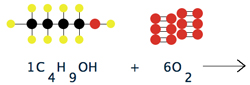# Balancing Chemical EquationsIn chemical reactions, sets of compounds interact with each other to form new compounds. Chemists use equations to describe these interactions. Like mathematical equations, chemical equations conform to a set of rules. This allows equations to provide detailed information about a reaction.

A chemical equation can be thought of as a recipe for making a set of chemical compound using other compounds as starting material. A properly formed chemical equation contains:

• Reactants - the substances transformed by the reaction. These go on the left.
• Products - the substances formed by the reaction. These go on the right.
• An arrow separates reactants from products.
• In reactions with more than one reactant or product, plus signs separate the individual products and reactants from each other.
• Numbers in front of each compound specify how many of each is required to convert all of the reactants to products.
The chemical equation for the formation of sugar from water and carbon dioxide is:

6CO2 + 6H2O -> C6H12O6 + 6O2

Properly written, the equation obey's the law of conservation of mass*. The law states that the mass of the reactants going into a reaction must be equal to the mass of the products. This mean that nothing can be gained or lost in the process. A chemical reaction involves the rearrangement of atoms between molecules, not the creation or destruction of atoms.

To make sure that the equation conforms to the law of conservation of mass the equation must be balanced. A balanced chemical equation is one where there are the same number of atoms of each element on either side of the equation. This is the significance of the numbers written before each compound in the reaction. Without the proper number of reactants and products, a chemical equation is not a complete representation of the reaction.

The process of balancing an equation involves adding to each side of the equation until there are the same number of atoms of each element present on both sides.

The illustration explores the process of balancing a chemical equation using the oxidation of a number of different hydrocarbons containing between one and five carbon atoms. A video demonstration of the illustration can be found below.

Video Overview:

Related Content